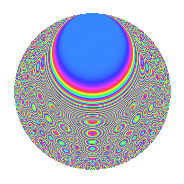# Properties

 Label 224.3.wLevel 224 Weight 3 Character orbit w Rep. character $$\chi_{224}(43,\cdot)$$ Character field $$\Q(\zeta_{8})$$ Dimension 192 Newform subspaces 1 Sturm bound 96 Trace bound 0

# Related objects

## Defining parameters

 Level: $$N$$ $$=$$ $$224 = 2^{5} \cdot 7$$ Weight: $$k$$ $$=$$ $$3$$ Character orbit: $$[\chi]$$ $$=$$ 224.w (of order $$8$$ and degree $$4$$) Character conductor: $$\operatorname{cond}(\chi)$$ $$=$$ $$32$$ Character field: $$\Q(\zeta_{8})$$ Newform subspaces: $$1$$ Sturm bound: $$96$$ Trace bound: $$0$$

## Dimensions

The following table gives the dimensions of various subspaces of $$M_{3}(224, [\chi])$$.

Total New Old
Modular forms 264 192 72
Cusp forms 248 192 56
Eisenstein series 16 0 16

## Trace form

 $$192q + O(q^{10})$$ $$192q + 80q^{10} + 96q^{12} - 20q^{16} - 60q^{18} - 260q^{22} + 64q^{23} - 144q^{24} - 200q^{26} + 192q^{27} - 40q^{30} + 40q^{32} + 120q^{34} + 464q^{36} + 504q^{38} - 384q^{39} + 360q^{40} - 96q^{43} + 52q^{44} + 64q^{46} - 104q^{48} - 312q^{50} - 384q^{51} - 320q^{52} + 160q^{53} - 576q^{54} - 512q^{55} - 196q^{56} - 360q^{58} - 872q^{60} + 128q^{61} - 408q^{62} + 832q^{66} + 160q^{67} + 856q^{68} - 384q^{69} + 336q^{70} + 1488q^{72} + 308q^{74} + 768q^{75} + 1024q^{76} - 224q^{77} - 408q^{78} + 1024q^{79} - 1040q^{80} - 240q^{82} - 1384q^{86} + 896q^{87} - 560q^{88} - 1320q^{90} - 380q^{92} - 936q^{94} - 1088q^{96} - 512q^{99} + O(q^{100})$$

## Decomposition of $$S_{3}^{\mathrm{new}}(224, [\chi])$$ into newform subspaces

Label Dim. $$A$$ Field CM Traces $q$-expansion
$$a_2$$ $$a_3$$ $$a_5$$ $$a_7$$
224.3.w.a $$192$$ $$6.104$$ None $$0$$ $$0$$ $$0$$ $$0$$

## Decomposition of $$S_{3}^{\mathrm{old}}(224, [\chi])$$ into lower level spaces

$$S_{3}^{\mathrm{old}}(224, [\chi]) \cong$$ $$S_{3}^{\mathrm{new}}(32, [\chi])$$$$^{\oplus 2}$$

## Hecke characteristic polynomials

There are no characteristic polynomials of Hecke operators in the database